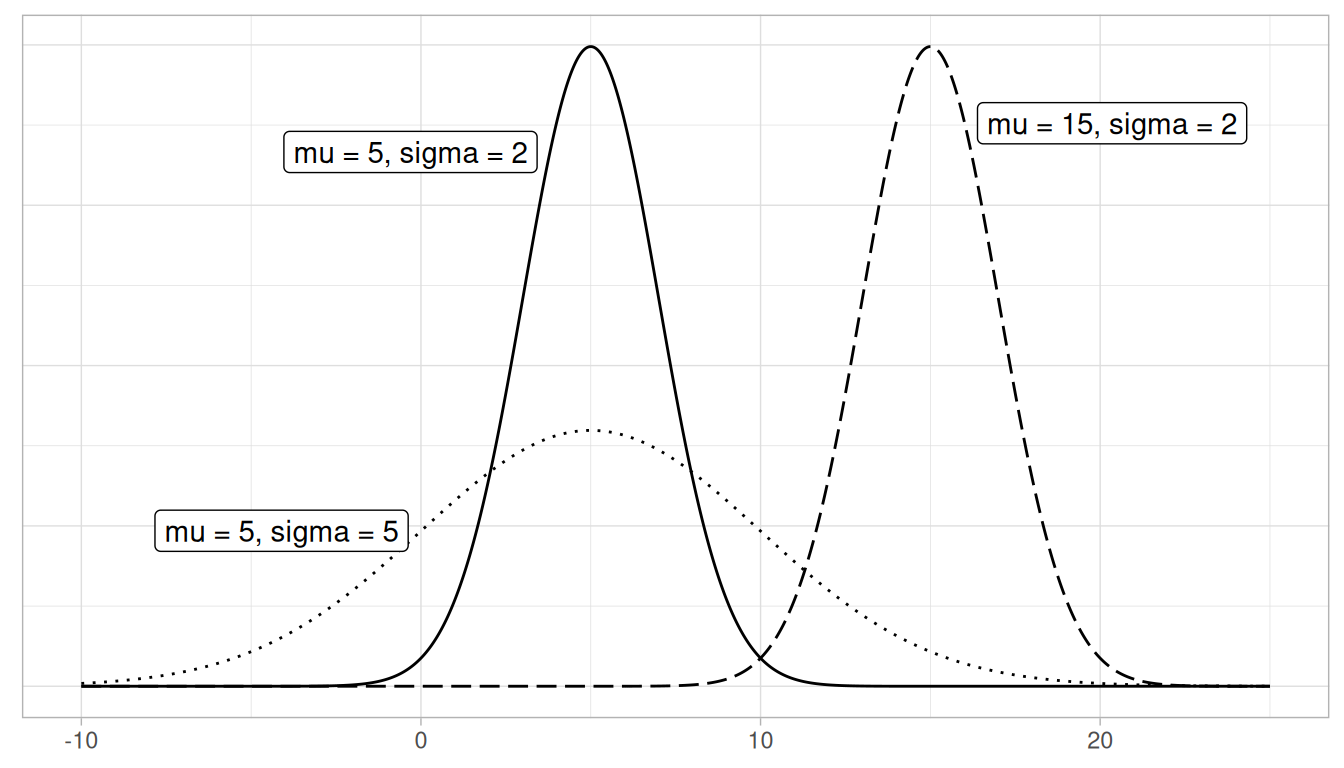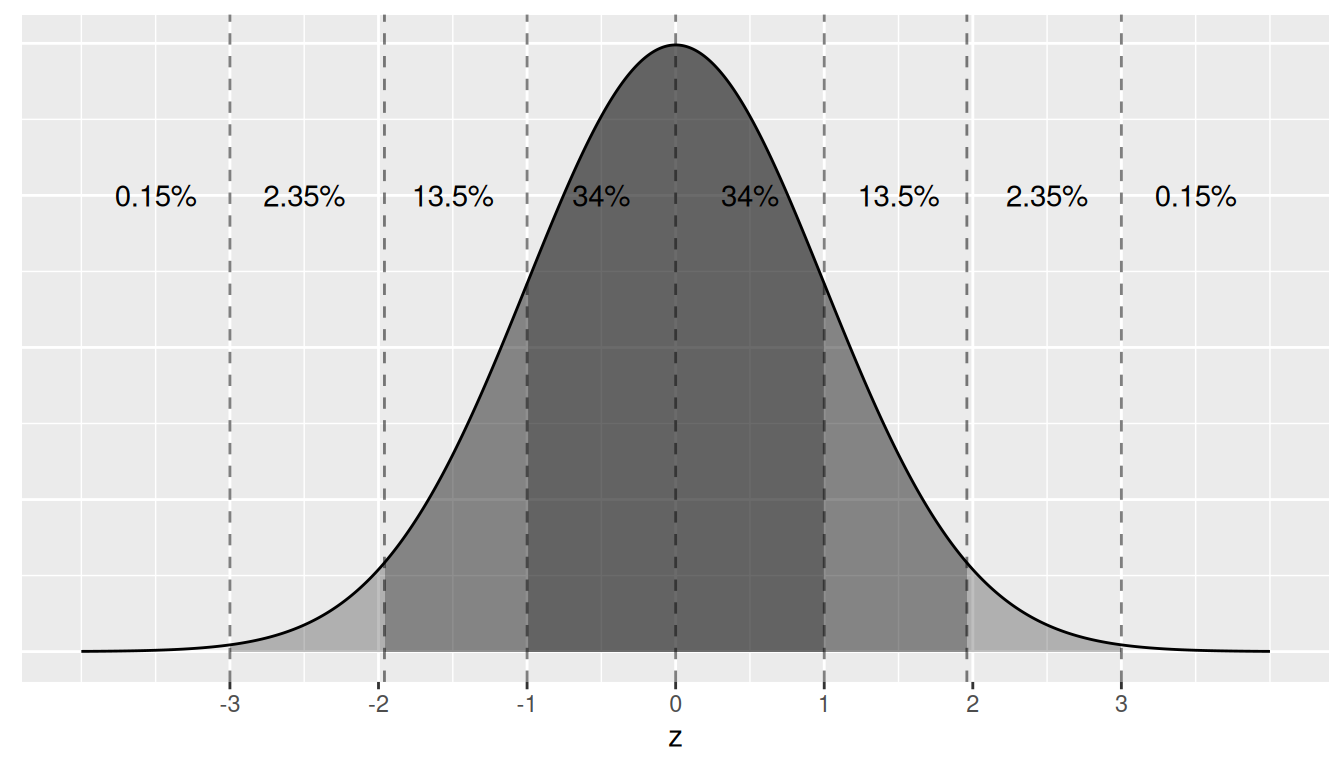## A.2 Normal distribution

Let’s next discuss one particular kind of distribution: normal distributions. Such bell-shaped distributions are defined by two values: (1) the mean $$\mu$$ (“mu”) which locates the center of the distribution and (2) the standard deviation $$\sigma$$ (“sigma”) which determines the variation of the distribution. In Figure A.1, we plot three normal distributions where:

1. The solid normal curve has mean $$\mu = 5$$ & standard deviation $$\sigma = 2$$.
2. The dotted normal curve has mean $$\mu = 5$$ & standard deviation $$\sigma = 5$$.
3. The dashed normal curve has mean $$\mu = 15$$ & standard deviation $$\sigma = 2$$.FIGURE A.1: Three normal distributions.

Notice how the solid and dotted line normal curves have the same center due to their common mean $$\mu$$ = 5. However, the dotted line normal curve is wider due to its larger standard deviation of $$\sigma$$ = 5. On the other hand, the solid and dashed line normal curves have the same variation due to their common standard deviation $$\sigma$$ = 2. However, they are centered at different locations.

When the mean $$\mu$$ = 0 and the standard deviation $$\sigma$$ = 1, the normal distribution has a special name. It’s called the standard normal distribution or the $$z$$-curve.

Furthermore, if a variable follows a normal curve, there are three rules of thumb we can use:

1. 68% of values will lie within $$\pm$$ 1 standard deviation of the mean.
2. 95% of values will lie within $$\pm$$ 1.96 $$\approx$$ 2 standard deviations of the mean.
3. 99.7% of values will lie within $$\pm$$ 3 standard deviations of the mean.

Let’s illustrate this on a standard normal curve in Figure A.2. The dashed lines are at -3, -1.96, -1, 0, 1, 1.96, and 3. These 7 lines cut up the x-axis into 8 segments. The areas under the normal curve for each of the 8 segments are marked and add up to 100%. For example:

1. The middle two segments represent the interval -1 to 1. The shaded area above this interval represents 34% + 34% = 68% of the area under the curve. In other words, 68% of values.
2. The middle four segments represent the interval -1.96 to 1.96. The shaded area above this interval represents 13.5% + 34% + 34% + 13.5% = 95% of the area under the curve. In other words, 95% of values.
3. The middle six segments represent the interval -3 to 3. The shaded area above this interval represents 2.35% + 13.5% + 34% + 34% + 13.5% + 2.35% = 99.7% of the area under the curve. In other words, 99.7% of values.FIGURE A.2: Rules of thumb about areas under normal curves.

Learning check

Say you have a normal distribution with mean $$\mu = 6$$ and standard deviation $$\sigma = 3$$.

(LCA.1) What proportion of the area under the normal curve is less than 3? Greater than 12? Between 0 and 12?

(LCA.2) What is the 2.5th percentile of the area under the normal curve? The 97.5th percentile? The 100th percentile?